# RegularGrid¶

class RegularGrid(*args)

Regular Grid.

Available constructors:

RegularGrid(start, step, n)

RegularGrid(mesh)

Parameters:
startfloat

The start time stamp of the grid.

stepfloat, positive

The step between to consecutive time stamps.

nint

The number of time stamps in the grid, including the start and the end time stamps.

meshMesh

The mesh must be in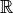, regular and sorted in the increasing order.

Notes

The time stamps of the regular grid are: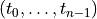where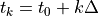for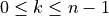and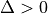the step.

Examples

>>> import openturns as ot
>>> myRegularGrid = ot.RegularGrid(0.0, 0.1, 100)


Methods

 ImportFromMSHFile(fileName) Import mesh from FreeFem 2-d mesh files. checkPointInSimplexWithCoordinates(point, index) Check if a point is inside a simplex and returns its barycentric coordinates. Compute the P1 Lagrange finite element gram matrix of the mesh. Compute the volume of all simplices. Compute an approximation of an integral defined over the mesh. Draw the mesh. Draw the mesh of dimension 1. Draw the mesh of dimension 2. draw3D(*args) Draw the bidimensional projection of the mesh. exportToVTKFile(*args) Export the mesh to a VTK file. Make all the simplices positively oriented. follows(starter) Check if the given grid follows the current one. Accessor to the object's name. Description accessor. Dimension accessor. Accessor to the first time stamp after the last time stamp of the grid. Accessor to the object's id. Lower bound accessor. Accessor to the number of time stamps in the grid. Accessor to the object's name. Accessor to the object's shadowed id. getSimplex(index) Get the simplex of a given index. Get the simplices of the mesh. Get the number of simplices of the mesh. Accessor to the start time stamp. Accessor to the step. Upper bound accessor. Accessor to the time stamps at a gien index. Accessor to all the time stamps. getVertex(index) Get the vertex of a given index. Get the vertices of the mesh. Get the number of vertices of the mesh. Accessor to the object's visibility state. Get the volume of the mesh. Test if the object is named. Test if the object has a distinguishable name. Check whether the mesh is empty. Check if the mesh is numerically empty. Check if the mesh is regular (only for 1-d meshes). Check the mesh validity. setDescription(description) Description accessor. setName(name) Accessor to the object's name. Accessor to the object's shadowed id. setSimplices(simplices) Set the simplices of the mesh. setVertex(index, vertex) Set a vertex of a given index. setVertices(vertices) Set the vertices of the mesh. setVisibility(visible) Accessor to the object's visibility state. streamToVTKFormat(*args) Give a VTK representation of the mesh.
__init__(*args)
static ImportFromMSHFile(fileName)

Import mesh from FreeFem 2-d mesh files.

Parameters:
MSHFilestr

A MSH ASCII file.

Returns:
meshMesh

Mesh defined in the file MSHFile.

checkPointInSimplexWithCoordinates(point, index)

Check if a point is inside a simplex and returns its barycentric coordinates.

Parameters:
pointsequence of float

Point of dimension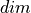, the dimension of the vertices of the mesh.

indexint

Integer characterizes one simplex of the mesh.

Returns:
isInsidebool

Flag telling whether point is inside the simplex of index index.

coordinatesPoint

The barycentric coordinates of the given point wrt the vertices of the simplex.

Notes

The tolerance for the check on the barycentric coordinates can be tweaked using the key Mesh-CoordinateEpsilon.

Examples

>>> import openturns as ot
>>> vertices = [[0.0, 0.0], [1.0, 0.0], [1.0, 1.0]]
>>> simplex = [[0, 1, 2]]
>>> mesh2d = ot.Mesh(vertices, simplex)
>>> # Create a point A inside the simplex
>>> pointA = [0.6, 0.3]
>>> print(mesh2d.checkPointInSimplexWithCoordinates(pointA, 0))
[True, class=Point name=Unnamed dimension=3 values=[0.4,0.3,0.3]]
>>> # Create a point B outside the simplex
>>> pointB = [1.1, 0.6]
>>> print(mesh2d.checkPointInSimplexWithCoordinates(pointB, 0))
[False, class=Point name=Unnamed dimension=3 values=[-0.1,0.5,0.6]]

computeP1Gram()

Compute the P1 Lagrange finite element gram matrix of the mesh.

Returns:
gramCovarianceMatrix

P1 Lagrange finite element gram matrix of the mesh.

Notes

The P1 Lagrange finite element space associated to a mesh with vertices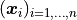is the space of piecewise-linear functions generated by the functions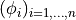, where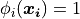,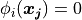for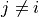and the restriction of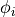to any simplex is an affine function. The vertices that are not included into at least one simplex are not taken into account.

The gram matrix of the mesh is defined as the symmetric positive definite matrix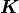whose generic element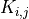is given by: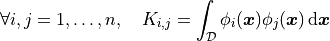This method is used in several algorithms related to stochastic process representation such as the Karhunen-Loeve decomposition.

Examples

>>> import openturns as ot
>>> # Define the vertices of the mesh
>>> vertices = [[0.0, 0.0], [1.0, 0.0], [1.0, 1.0], [1.5, 1.0]]
>>> # Define the simplices of the mesh
>>> simplices = [[0, 1, 2], [1, 2, 3]]
>>> # Create the mesh of dimension 2
>>> mesh2d = ot.Mesh(vertices, simplices)
>>> print(mesh2d.computeP1Gram())
[[ 0.0833333 0.0416667 0.0416667 0         ]
[ 0.0416667 0.125     0.0625    0.0208333 ]
[ 0.0416667 0.0625    0.125     0.0208333 ]
[ 0         0.0208333 0.0208333 0.0416667 ]]

computeSimplicesVolume()

Compute the volume of all simplices.

Returns:
volumePoint

Volume of all simplices.

Examples

>>> import openturns as ot
>>> vertices = [[0.0, 0.0], [1.0, 0.0], [1.0, 1.0]]
>>> simplex = [[0, 1, 2]]
>>> mesh2d = ot.Mesh(vertices, simplex)
>>> print(mesh2d.computeSimplicesVolume())
[0.5]

computeWeights()

Compute an approximation of an integral defined over the mesh.

Returns:
weightsPoint

Weights such that an integral of a function over the mesh is a weighted sum of its values at the vertices.

draw()

Draw the mesh.

Returns:
graphGraph

If the dimension of the mesh is 1, it draws the corresponding interval, using the draw1D() method; if the dimension is 2, it draws the triangular simplices, using the draw2D() method; if the dimension is 3, it projects the simplices on the plane of the two first components, using the draw3D() method with its default parameters, superposing the simplices.

draw1D()

Draw the mesh of dimension 1.

Returns:
graphGraph

Draws the line linking the vertices of the mesh when the mesh is of dimension 1.

Examples

>>> import openturns as ot
>>> from openturns.viewer import View
>>> vertices = [[0.5], [1.5], [2.1], [2.7]]
>>> simplices = [[0, 1], [1, 2], [2, 3]]
>>> mesh1d = ot.Mesh(vertices, simplices)
>>> # Create a graph
>>> aGraph = mesh1d.draw1D()
>>> # Draw the mesh
>>> View(aGraph).show()

draw2D()

Draw the mesh of dimension 2.

Returns:
graphGraph

Draws the edges of each simplex, when the mesh is of dimension 2.

Examples

>>> import openturns as ot
>>> from openturns.viewer import View
>>> vertices = [[0.0, 0.0], [1.0, 0.0], [1.0, 1.0], [1.5, 1.0]]
>>> simplices = [[0, 1, 2], [1, 2, 3]]
>>> mesh2d = ot.Mesh(vertices, simplices)
>>> # Create a graph
>>> aGraph = mesh2d.draw2D()
>>> # Draw the mesh
>>> View(aGraph).show()

draw3D(*args)

Draw the bidimensional projection of the mesh.

Available usages:

draw3D(drawEdge=True, thetaX=0.0, thetaY=0.0, thetaZ=0.0, shading=False, rho=1.0)

Parameters:
drawEdgebool

Tells if the edge of each simplex has to be drawn.

thetaXfloat

Gives the value of the rotation along the X axis in radian.

thetaYfloat

Gives the value of the rotation along the Y axis in radian.

thetaZfloat

Gives the value of the rotation along the Z axis in radian.

rotationSquareMatrix

Operates a rotation on the mesh before its projection of the plane of the two first components.

Enables to give a visual perception of depth and orientation.

rhofloat,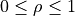Contraction factor of the simplices. If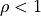, all the simplices are contracted and appear deconnected: some holes are created, which enables to see inside the mesh. If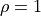, the simplices keep their initial size and appear connected. If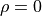, each simplex is reduced to its gravity center.

Returns:
graphGraph

Draws the bidimensional projection of the mesh on the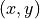plane.

Examples

>>> import openturns as ot
>>> from openturns.viewer import View
>>> from math import cos, sin, pi
>>> vertices = [[0.0, 0.0, 0.0], [0.0, 0.0, 1.0], [0.0, 1.0, 0.0],
...             [0.0, 1.0, 1.0], [1.0, 0.0, 0.0], [1.0, 0.0, 1.0],
...             [1.0, 1.0, 0.0], [1.0, 1.0, 1.0]]
>>> simplices = [[0, 1, 2, 4], [3, 5, 6, 7],[1, 2, 3, 6],
...              [1, 2, 4, 6], [1, 3, 5, 6], [1, 4, 5, 6]]
>>> mesh3d = ot.Mesh(vertices, simplices)
>>> # Create a graph
>>> aGraph = mesh3d.draw3D()
>>> # Draw the mesh
>>> View(aGraph).show()
>>> rotation = ot.SquareMatrix(3)
>>> rotation[0, 0] = cos(pi / 3.0)
>>> rotation[0, 1] = sin(pi / 3.0)
>>> rotation[1, 0] = -sin(pi / 3.0)
>>> rotation[1, 1] = cos(pi / 3.0)
>>> rotation[2, 2] = 1.0
>>> # Create a graph
>>> aGraph = mesh3d.draw3D(True, rotation, True, 1.0)
>>> # Draw the mesh
>>> View(aGraph).show()

exportToVTKFile(*args)

Export the mesh to a VTK file.

Parameters:
myVTKFile.vtkstr

Name of the created file which contains the mesh and the associated random values that can be visualized with the open source software Paraview.

fixOrientation()

Make all the simplices positively oriented.

Examples

>>> import openturns as ot
>>> vertices = [[0.0, 0.0], [1.0, 0.0], [1.0, 1.0]]
>>> simplex = [[0, 2, 1]]
>>> mesh2d = ot.Mesh(vertices, simplex)
>>> print(mesh2d)
class=Mesh name=Unnamed dimension=2 vertices=class=Sample name=Unnamed implementation=class=SampleImplementation name=Unnamed size=3 dimension=2 data=[[0,0],[1,0],[1,1]] simplices=[[0,2,1]]
>>> mesh2d.fixOrientation()
>>> print(mesh2d)
class=Mesh name=Unnamed dimension=2 vertices=class=Sample name=Unnamed implementation=class=SampleImplementation name=Unnamed size=3 dimension=2 data=[[0,0],[1,0],[1,1]] simplices=[[2,0,1]]

follows(starter)

Check if the given grid follows the current one.

Parameters:
newGridRegularGrid

A new regular grid.

Returns:

The answer is True if the newGrid directly follows the current one.

getClassName()

Accessor to the object’s name.

Returns:
class_namestr

The object class name (object.__class__.__name__).

getDescription()

Description accessor.

Returns:
descriptionDescription

Description of the vertices.

Examples

>>> import openturns as ot
>>> mesh = ot.Mesh()
>>> vertices = ot.Sample([[0.0, 0.0], [1.0, 0.0], [1.0, 1.0]])
>>> vertices.setDescription(['X', 'Y'])
>>> mesh.setVertices(vertices)
>>> print(mesh.getDescription())
[X,Y]

getDimension()

Dimension accessor.

Returns:
dimensionint

Dimension of the vertices.

getEnd()

Accessor to the first time stamp after the last time stamp of the grid.

Returns:
endPointfloat

The first point that follows the last point of the grid: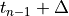. The end point is not in the grid.

getId()

Accessor to the object’s id.

Returns:
idint

Internal unique identifier.

getLowerBound()

Lower bound accessor.

Returns:
lower_boundPoint

Min of the vertices.

getN()

Accessor to the number of time stamps in the grid.

Returns:
nint

The number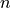of time stamps in the grid.

getName()

Accessor to the object’s name.

Returns:
namestr

The name of the object.

Accessor to the object’s shadowed id.

Returns:
idint

Internal unique identifier.

getSimplex(index)

Get the simplex of a given index.

Parameters:
indexint

Index characterizing one simplex of the mesh.

Returns:
indicesIndices

Indices defining the simplex of index index. The simplex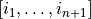relies the vertices of index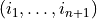in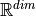. In dimension 1, a simplex is an interval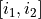; in dimension 2, it is a triangle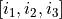.

Examples

>>> import openturns as ot
>>> vertices = [[0.0, 0.0], [1.0, 0.0], [1.0, 1.0], [1.5, 1.0]]
>>> simplices = [[0, 1, 2], [1, 2, 3]]
>>> mesh2d = ot.Mesh(vertices, simplices)
>>> print(mesh2d.getSimplex(0))
[0,1,2]
>>> print(mesh2d.getSimplex(1))
[1,2,3]

getSimplices()

Get the simplices of the mesh.

Returns:
indicesCollectioncollection of Indices

List of indices defining all the simplices. The simplexrelies the vertices of indexin. In dimension 1, a simplex is an interval; in dimension 2, it is a triangle.

Examples

>>> import openturns as ot
>>> vertices = [[0.0, 0.0], [1.0, 0.0], [1.0, 1.0], [1.5, 1.0]]
>>> simplices = [[0, 1, 2], [1, 2, 3]]
>>> mesh2d = ot.Mesh(vertices, simplices)
>>> print(mesh2d.getSimplices())
[[0,1,2],[1,2,3]]

getSimplicesNumber()

Get the number of simplices of the mesh.

Returns:
numberint

Number of simplices of the mesh.

getStart()

Accessor to the start time stamp.

Returns:
startfloat

The start point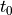of the grid.

getStep()

Accessor to the step.

Returns:
stepfloat

The step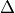between two consecutive time stamps.

getUpperBound()

Upper bound accessor.

Returns:
upper_boundPoint

Max of the vertices.

getValue(i)

Accessor to the time stamps at a gien index.

Parameters:
kint,.

Index of a time stamp.

Returns:
valuefloat

The time stamp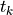.

getValues()

Accessor to all the time stamps.

Returns:
valuesPoint

The collection of the time stamps.

getVertex(index)

Get the vertex of a given index.

Parameters:
indexint

Index characterizing one vertex of the mesh.

Returns:
vertexPoint

Coordinates inof the vertex of index index, whereis the dimension of the vertices of the mesh.

Examples

>>> import openturns as ot
>>> vertices = [[0.0, 0.0], [1.0, 0.0], [1.0, 1.0]]
>>> simplices = [[0, 1, 2]]
>>> mesh2d = ot.Mesh(vertices, simplices)
>>> print(mesh2d.getVertex(1))
[1,0]
>>> print(mesh2d.getVertex(0))
[0,0]

getVertices()

Get the vertices of the mesh.

Returns:
verticesSample

Coordinates inof the vertices, whereis the dimension of the vertices of the mesh.

Examples

>>> import openturns as ot
>>> vertices = [[0.0, 0.0], [1.0, 0.0], [1.0, 1.0]]
>>> simplices = [[0, 1, 2]]
>>> mesh2d = ot.Mesh(vertices, simplices)
>>> print(mesh2d.getVertices())
0 : [ 0 0 ]
1 : [ 1 0 ]
2 : [ 1 1 ]

getVerticesNumber()

Get the number of vertices of the mesh.

Returns:
numberint

Number of vertices of the mesh.

getVisibility()

Accessor to the object’s visibility state.

Returns:
visiblebool

Visibility flag.

getVolume()

Get the volume of the mesh.

Returns:
volumefloat

Geometrical volume of the mesh which is the sum of its simplices’ volumes.

Examples

>>> import openturns as ot
>>> vertices = [[0.0, 0.0], [1.0, 0.0], [1.0, 1.0], [1.5, 1.0]]
>>> simplices = [[0, 1, 2], [1, 2, 3]]
>>> mesh2d = ot.Mesh(vertices, simplices)
>>> mesh2d.getVolume()
0.75

hasName()

Test if the object is named.

Returns:
hasNamebool

True if the name is not empty.

hasVisibleName()

Test if the object has a distinguishable name.

Returns:
hasVisibleNamebool

True if the name is not empty and not the default one.

isEmpty()

Check whether the mesh is empty.

Returns:
emptybool

Tells if the mesh is empty, ie if its volume is null.

isNumericallyEmpty()

Check if the mesh is numerically empty.

Returns:
isEmptybool

Flag telling whether the mesh is numerically empty, i.e. if its numerical volume is inferior or equal to(defined in the ResourceMap:= Domain-SmallVolume).

Examples

>>> import openturns as ot
>>> vertices = [[0.0, 0.0], [1.0, 0.0], [1.0, 1.0]]
>>> simplex = [[0, 1, 2]]
>>> mesh2d = ot.Mesh(vertices, simplex)
>>> print(mesh2d.isNumericallyEmpty())
False

isRegular()

Check if the mesh is regular (only for 1-d meshes).

Returns:
isRegularbool

Tells if the mesh is regular or not.

Examples

>>> import openturns as ot
>>> vertices = [[0.5], [1.5], [2.4], [3.5]]
>>> simplices = [[0, 1], [1, 2], [2, 3]]
>>> mesh1d = ot.Mesh(vertices, simplices)
>>> print(mesh1d.isRegular())
False
>>> vertices = [[0.5], [1.5], [2.5], [3.5]]
>>> mesh1d = ot.Mesh(vertices, simplices)
>>> print(mesh1d.isRegular())
True

isValid()

Check the mesh validity.

Returns:
validitybool

Tells if the mesh is valid i.e. if there is non-overlaping simplices, no unused vertex, no simplices with duplicate vertices and no coincident vertices.

setDescription(description)

Description accessor.

Parameters:
descriptionsequence of str

Description of the vertices.

Examples

>>> import openturns as ot
>>> mesh = ot.Mesh()
>>> vertices = ot.Sample([[0.0, 0.0], [1.0, 0.0], [1.0, 1.0]])
>>> mesh.setVertices(vertices)
>>> mesh.setDescription(['X', 'Y'])
>>> print(mesh.getDescription())
[X,Y]

setName(name)

Accessor to the object’s name.

Parameters:
namestr

The name of the object.

Accessor to the object’s shadowed id.

Parameters:
idint

Internal unique identifier.

setSimplices(simplices)

Set the simplices of the mesh.

Parameters:
indices2-d sequence of int

List of indices defining all the simplices. The simplexrelies the vertices of indexin. In dimension 1, a simplex is an interval; in dimension 2, it is a triangle.

Examples

>>> import openturns as ot
>>> mesh = ot.Mesh()
>>> simplices = [[0, 1, 2], [1, 2, 3]]
>>> mesh.setSimplices(simplices)

setVertex(index, vertex)

Set a vertex of a given index.

Parameters:
indexint

Index of the vertex to set.

vertexsequence of float

Cordinates inof the vertex of index index, whereis the dimension of the vertices of the mesh.

Examples

>>> import openturns as ot
>>> vertices = [[0.0, 0.0], [1.0, 0.0], [1.0, 1.0]]
>>> simplices = [[0, 1, 2]]
>>> mesh = ot.Mesh(vertices, simplices)
>>> vertex = [0.0, 0.5]
>>> mesh.setVertex(0, vertex)
>>> print(mesh.getVertices())
0 : [ 0   0.5 ]
1 : [ 1   0   ]
2 : [ 1   1   ]

setVertices(vertices)

Set the vertices of the mesh.

Parameters:
vertices2-d sequence of float

Cordinates inof the vertices, whereis the dimension of the vertices of the mesh.

Examples

>>> import openturns as ot
>>> mesh = ot.Mesh()
>>> vertices = [[0.0, 0.0], [1.0, 0.0], [1.0, 1.0]]
>>> mesh.setVertices(vertices)

setVisibility(visible)

Accessor to the object’s visibility state.

Parameters:
visiblebool

Visibility flag.

streamToVTKFormat(*args)

Give a VTK representation of the mesh.

Returns:
streamstr

VTK representation of the mesh.

## Examples using the class¶Kolmogorov-Smirnov : understand the p-value

Kolmogorov-Smirnov : understand the p-valueKolmogorov-Smirnov : get the statistics distribution

Kolmogorov-Smirnov : get the statistics distributionEstimate a multivariate ARMA process

Estimate a multivariate ARMA processEstimate a non stationary covariance function

Estimate a non stationary covariance functionEstimate a scalar ARMA process

Estimate a scalar ARMA processEstimate a spectral density function

Estimate a spectral density functionEstimate a stationary covariance function

Estimate a stationary covariance functionCreate a functional basis process

Create a functional basis processAdd a trend to a process

Add a trend to a processCreate a gaussian process from a cov. model using HMatrix

Create a gaussian process from a cov. model using HMatrixCreate a white noise process

Create a white noise processCreate a discrete Markov chain process

Create a discrete Markov chain processManipulate a time series

Manipulate a time seriesCreate a stationary covariance model

Create a stationary covariance modelCreate a normal process

Create a normal processCreate a random walk process

Create a random walk processCreate a spectral model

Create a spectral modelCreate a process from random vectors and processes

Create a process from random vectors and processesSample trajectories from a Gaussian Process with correlated outputs

Sample trajectories from a Gaussian Process with correlated outputsDraw fields

Draw fieldsCreate and manipulate an ARMA process

Create and manipulate an ARMA processCompare covariance models

Compare covariance modelsCreate a mesh

Create a meshOver-fitting and model selection

Over-fitting and model selectionKriging : generate trajectories from a metamodel

Kriging : generate trajectories from a metamodelKriging : quick-start

Kriging : quick-startSequentially adding new points to a kriging

Sequentially adding new points to a krigingKriging : choose a trend vector space

Kriging : choose a trend vector spaceKriging : draw covariance models

Kriging : draw covariance modelsCreate a threshold event

Create a threshold eventTime variant system reliability problem

Time variant system reliability problemEstimate a process-based event probability

Estimate a process-based event probabilityEstimate Sobol indices on a field to point function

Estimate Sobol indices on a field to point functionValue function

Value functionVertex value function

Vertex value functionLogistic growth model

Logistic growth modelGenerate flooding model observations

Generate flooding model observationsGenerate observations of the Chaboche mechanical model

Generate observations of the Chaboche mechanical modelBayesian calibration of a computer code

Bayesian calibration of a computer codeCompute confidence intervals of a univariate noisy function

Compute confidence intervals of a univariate noisy functionHow to fill an area

How to fill an areaA quick start guide to graphs

A quick start guide to graphs